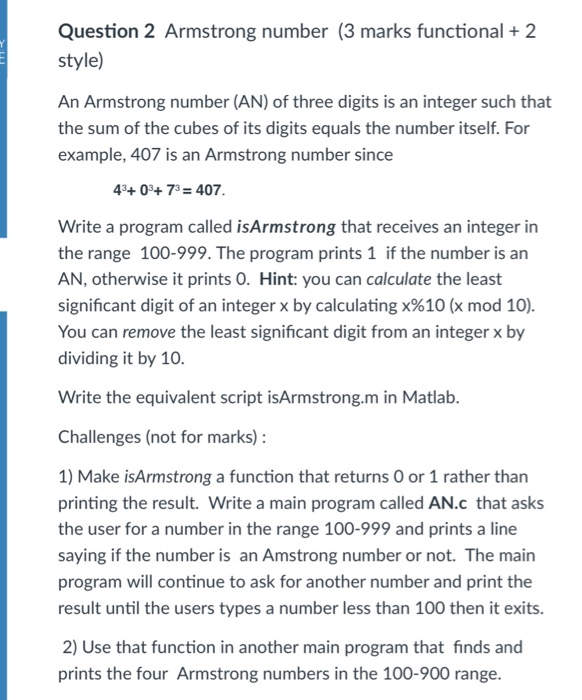# Question & Answer: An Armstrong number (AN) of three digits is an integer such that the sum of the cubes of its digits equals the number itself. For example, 407 is an Ar…..An Armstrong number (AN) of three digits is an integer such that the sum of the cubes of its digits equals the number itself. For example, 407 is an Armstrong number since 4^3 + 0^3 + 7^3 = 407. Write a program called isArmstrong that receives an integer in the range 100-999. The program prints 1 if the number is an AN, otherwise it prints 0. You can remove the least significant digit from an integer x by dividing it by 10. Write the equivalent script isArmstrong.m in Matlab. Challenges (not for marks): Make isArmstrong a function that returns 0 or 1 rather than printing the result. Write a main program called AN.c that asks the user for a number in the range 100-999 and prints a line saying if the number is an Amstrong number or not. The main program will continue to ask for another number and print the result until the users types a number less than 100 then it exits. Use that function in another main program that finds and prints the four Armstrong numbers in the 100-900 range.

Solution:

Don't use plagiarized sources. Get Your Custom Essay on
Question & Answer: An Armstrong number (AN) of three digits is an integer such that the sum of the cubes of its digits equals the number itself. For example, 407 is an Ar…..
GET AN ESSAY WRITTEN FOR YOU FROM AS LOW AS \$13/PAGE

isArmstrong.c

#include<stdio.h>

int main()

{

int num;

// Enter number

printf(“Enter the number in the range 100-999: “);

scanf(“%d”,&num);

int val=num;

int arm=0;

do

{

// Take least significant digit and add by cubing it

arm=arm+((num%10)*(num%10)*(num%10));

// Divide the number by 10

num=num/10;

}while(num!=0);

// If the number equals arm

if(val==arm)

// Number is armstrong

printf(“1”);

else

// Number is not armstrong

printf(“0”);

getchar();

getchar();

}

Output:

Enter the number in the range 100-999: 1

1

isArmstrong.m

num=input(‘Enter the number in the range 100-999: ‘);

% Convert number to string

num_str=num2str(num);

tot=0;

for i=1:length(num_str)

% convert each character to number

v=str2num(num_str(i));

% Find the cube

tot=tot+v*v*v;

end

% Check number is armstrong

if(tot==num)

disp(‘1’)

else

disp(‘0’)

end

Output:

Enter the number in the range 100-999: 407

1

Challenges:

1)

AN.c

#include<stdio.h>

int isArmstrong(int num)

{

int val=num;

int arm=0;

do

{

// Take least significant digit and add by cubing it

arm=arm+((num%10)*(num%10)*(num%10));

// Divide the number by 10

num=num/10;

}while(num!=0);

// If the number equals arm

if(val==arm)

// Number is armstrong

return 1;

else

// Number is not armstrong

return 0;

}

int main()

{

int num;

while(true)

{

// Enter number

printf(“Enter the number in the range 100-999: “);

scanf(“%d”,&num);

if(num<100)

break;

if(isArmstrong(num)==1)

// Number is armstrong

printf(“1n”);

else

// Number is not armstrong

printf(“0n”);

};

getchar();

getchar();

}

Output:

Enter the number in the range 100-999: 407

1

Enter the number in the range 100-999: 253

0

Enter the number in the range 100-999: 98

2)

#include<stdio.h>

int isArmstrong(int num)

{

int val=num;

int arm=0;

do

{

// Take least significant digit and add by cubing it

arm=arm+((num%10)*(num%10)*(num%10));

// Divide the number by 10

num=num/10;

}while(num!=0);

// If the number equals arm

if(val==arm)

// Number is armstrong

return 1;

else

// Number is not armstrong

return 0;

}

int main()

{

int num;

num=100;

int i=0;

while(i<5)

{

if(isArmstrong(num)==1)

{

// Number is armstrong

printf(“%dn”,num);;

i++;

}

num++;

};

getchar();

getchar();

}

Output:

153

370

371

407